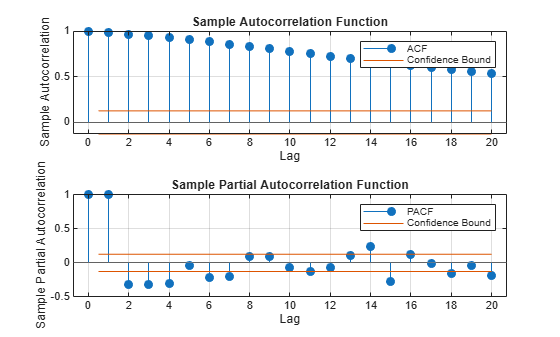# Estimate a Regression Model with ARIMA Errors

This example shows how to estimate the sensitivity of the US Gross Domestic Product (GDP) to changes in the Consumer Price Index (CPI) using estimate.

Load the US macroeconomic data set, Data_USEconModel. Plot the GDP and CPI.

load Data_USEconModel gdp = DataTable.GDP; cpi = DataTable.CPIAUCSL; figure plot(dates,gdp) title('{\bf US Gross Domestic Product, Q1 in 1947 to Q1 in 2009}') datetick axis tightfigure plot(dates,cpi) title('{\bf US Consumer Price Index, Q1 in 1947 to Q1 in 2009}') datetick axis tightgdp and cpi seem to increase exponentially.

Regress gdp onto cpi. Plot the residuals.

XDes = [ones(length(cpi),1) cpi]; % Design matrix beta = XDes\gdp; u = gdp - XDes*beta; % Residuals figure plot(u) h1 = gca; hold on plot(h1.XLim,[0 0],'r:') title('{\bf Residual Plot}') hold offThe pattern of the residuals suggests that the standard linear model assumption of uncorrelated errors is violated. The residuals appear autocorrelated.

Plot correlograms for the residuals.

figure subplot(2,1,1) autocorr(u) subplot(2,1,2) parcorr(u)The autocorrelation function suggests that the residuals are a nonstationary process.

Apply the first difference to the logged series to stabilize the residuals.

dlGDP = diff(log(gdp)); dlCPI = diff(log(cpi)); dlXDes = [ones(length(dlCPI),1) dlCPI]; beta = dlXDes\dlGDP; u = dlGDP - dlXDes*beta; figure plot(u); h2 = gca; hold on plot(h2.XLim,[0 0],'r:') title('{\bf Residual Plot, Transformed Series}') hold offfigure subplot(2,1,1) autocorr(u) subplot(2,1,2) parcorr(u)The residual plot from the transformed data suggests stabilized, albeit heteroscedastic, unconditional disturbances. The correlograms suggest that the unconditional disturbances follow an AR(1) process.

Specify the regression model with AR(1) errors:

$\begin{array}{c}\text{dlGDP}=\text{Intercept}+\text{dlCPI}\beta +{u}_{t}\\ {u}_{t}=\varphi {u}_{t-1}+{\epsilon }_{t}.\end{array}$

Mdl = regARIMA('ARLags',1);

estimate estimates any parameter having a value of NaN.

Fit Mdl to the data.

EstMdl = estimate(Mdl,dlGDP,'X',dlCPI,'Display','params');
 Regression with ARMA(1,0) Error Model (Gaussian Distribution): Value StandardError TStatistic PValue __________ _____________ __________ __________ Intercept 0.012762 0.0013472 9.4734 2.7098e-21 AR{1} 0.38245 0.052494 7.2856 3.2031e-13 Beta(1) 0.3989 0.077286 5.1614 2.4516e-07 Variance 9.0101e-05 5.947e-06 15.151 7.5075e-52 

Alternatively, estimate the regression coefficients and Newey-West standard errors using hac.

hac(dlCPI,dlGDP,'intercept',true,'display','full');
Estimator type: HAC Estimation method: BT Bandwidth: 4.1963 Whitening order: 0 Effective sample size: 248 Small sample correction: on Coefficient Estimates: | Coeff SE ------------------------ Const | 0.0115 0.0012 x1 | 0.5421 0.1005 Coefficient Covariances: | Const x1 -------------------------- Const | 0.0000 -0.0001 x1 | -0.0001 0.0101 

The intercept estimates are close, but the regression coefficient estimates corresponding to dlCPI are not. This is because regARIMA explicitly models for the autocorrelation of the disturbances. hac estimates the coefficients using ordinary least squares, and returns standard errors that are robust to the residual autocorrelation and heteroscedasticity.

Assuming that the model is correct, the results suggest that an increase of one point in the CPI rate increases the GDP growth rate by 0.399 points. This effect is significant according to the t statistic.

From here, you can use forecast or simulate to obtain forecasts and forecast intervals for the GDP rate. You can also compare several models by computing their AIC statistics using aicbic.